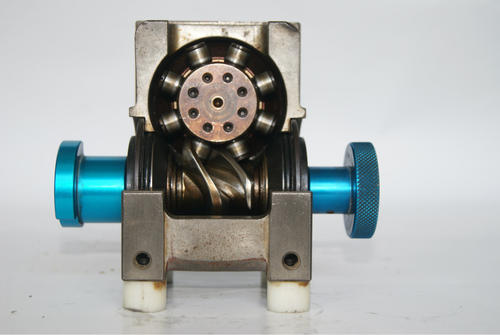分割器

# 分割器使用范畴计算方式举例详解

凸轮分割器的曲线特征：

凸轮曲线特性速度(Vm)、加速度(Am)、入力扭矩(Qm)对凸轮分割器机构的精度，寿命有着非常的的影响，选定适合的凸轮曲线，对机构而言，是一项非常重要的因素。

一般而言常用的凸轮曲线为变形正弦曲线(MS)、变形梯形曲线(MT)，变形等速度曲线(MCV)其选用原则如下：

使用范畴及计算

例一：适用于间歇回转圆盘

选用适当大小及规格之间歇分割器及所需动力之马达，请根据下列的内容计算，分割器计算设计

1. 间歇分割定位等分： N=6 S：分割数

2. 每等分回转时间：秒

3. 入力轴之回转数：n=80rpm：凸轮轴速度(每分钟回转数)

4. 凸轮曲线：修正正弦曲线

5. 回转盘之尺寸：O300X20t

6. 夹具之重量：3kg/组

7. 工作之重量：0.25kg/组

8. 转盘依靠其底部之滑动面支持本身重量负荷，有效 半径：R=100(m/m)

9. 夹具固定于节圆直径O200(m/m)处

解答 1~1 间歇分割定位等分：N=6 1~2 回转时间和定位时间之比为1:2，因此转位角度(INDEX ANGLE)，h=360°x1/1+2=120° 1~3 入力轴之回转数：n=80rpm 1~4 凸轮曲线系修正正弦曲线，因此Vm=1.76 Am=5.53 Qm=0.99 1~5 负载扭矩：Tt

1. 惯性扭矩：Tt

(a)转盘重量：W1 夹具重量：W2 工作重量：W3 则

W1=π/4x402x3x7.8x1/1000=29.405(kg)

W2=4x8=32(kg)

W3=0.5x8=4(kg)

(b)回转盘惯性矩：I1

夹具惯性矩：I2

工作惯性矩：I3

各为 I1=W1R2/2G=29.405x0.22/2x9.8=0.060(kg x m x s2)

I2=W2Re2/G=32x0.152/9.8=0.0753(kg x m x s2)

I3=W3Re2/G=4x0.152/9.8=0.0092(kg x m x s2)

(c)总惯性矩：I

I=I1+I2+I3 I=0.060+0.0735+0.0092=0.1426(kg x m x s2)(d)出力轴最大角加速度：a

(e)静扭矩(惯性扭矩)：Ti

Ti=1.a Ti=0.1426x 69.49=9.90.9(kgx m)

1-6实际负载扭矩：Te,安全负载之因数fc=1.5

Te=Tt.c=11.381x1.5=17.072(kg.m)

1-7入力轴扭矩：Tc,注：入力轴起动负载扭矩视为0，因此Tca=0

TC=360/H.NXQmxTe+Tca=360/120x8x0.99x17.072=0+6.334(kg.m)

1-8计算所需之马力：p

P=TCxn/716xj(hp)或p=tcxn/975xj(kw)

Thp=Tcxn/716xj(hp)或p= tcxn/975xj(kw)

假设效率j=60%

那么p=6.334x80/716x0.6=0.18(hp)或p=6.334x80/976x0.6=0.87(kw)

事实上。以上所计算之值为启动时最大马力，而连续传动所需之马力为1/2

2. 摩擦扭矩：Tf=μ

w.R=0.15×(29.405+32+4) ×0.15=1.472(Kg . m)

Tf=x

w x R=0.15x (11.026+18+1.5) x 0.1=0.458(kgx m)

3. 工作扭矩：Tw

在间歇分割时没有作功，因此Tw=0

4. 以上总负载扭矩：Tt

Tt=Ti+Tf+Tw=9.909+1.472+0=11.381(kgx m)

1~9选择适用之间歇分割器

根据以上所计算的资料以及入力轴之转数80rpm来选择、凡是出力轴扭矩高于以上所计算的Te值者均可选用。因为Te=17.072(kg-m),所以应采用RU-110DF型。